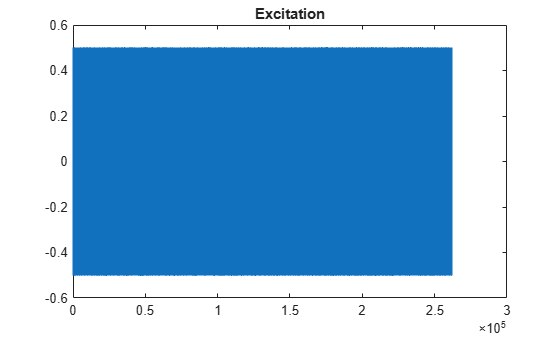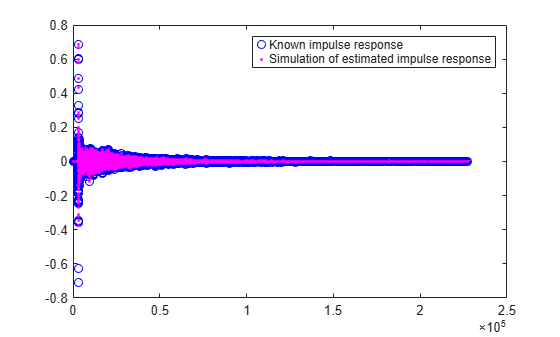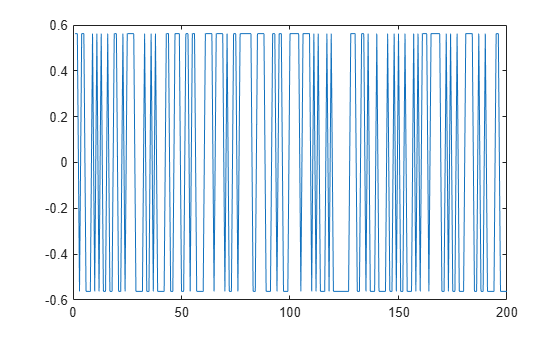# mls

Maximum length sequence

## Syntax

``excitation = mls``
``excitation = mls(L)``
``excitation = mls(L,Name,Value)``

## Description

````excitation = mls` returns an excitation signal generated using the maximum length sequence (MLS) technique. This type of sequence is a pseudo-random binary sequence.```

example

````excitation = mls(L)` specifies the output length `L` of the excitation signal.```

example

````excitation = mls(L,Name,Value)` specifies options using one or more `Name,Value` pair arguments, in addition to the input arguments in the previous syntaxes.```

## Examples

collapse all

Use `audioread` to read in an impulse response recording. Create a `dsp.FrequencyDomainFIRFilter` object to perform frequency domain filtering using the known impulse response.

```[irKnown,fs] = audioread('ChurchImpulseResponse-16-44p1-mono-5secs.wav'); systemModel = dsp.FrequencyDomainFIRFilter(irKnown');```

Create an MLS excitation signal by using the `mls` function. The MLS excitation signal must be longer than the impulse response. Note that the length of the MLS excitation is extended to the next power of two minus one.

```excitation = mls(numel(irKnown)+1); plot(excitation) title('Excitation')```Replicate the excitation signal four times to measure the average of three measurements. The recording of the first MLS sequence does include all the impulse response information, so `impzest` discards it as a warmup run. Pad the excitation signal with zeros to account for the filter latency.

```numRuns = 4; excrep = repmat(excitation,numRuns,1); excrep = [excrep;zeros(numel(irKnown)+1,1)];```

Pass the excitation signal through the known filter and then add noise to model a real-word recording (system response). Cut the delay introduced at the beginning by the filter.

```rec = systemModel(excrep); rec = rec + 0.1*randn(size(rec)); rec = rec(numel(irKnown)+2:end,:); plot(rec) title('System Response')```In a real-world scenario, the MLS sequence is played back in the system under test while recording. The recording would be cut so that it begins at the moment the MLS sequence is picked-up and truncated to last the duration of the repeated sequence.

Pass the excitation signal and the system response to the `impzest` function to estimate the impulse response. Plot the known impulse response and the simulation of the estimated impulse response for comparison.

```irEstimate = impzest(excitation,rec); samples = 1:numel(irKnown); plot(samples,irEstimate(samples),'bo', ... samples,irKnown(samples),'m.') legend('Known impulse response','Simulation of estimated impulse response')```Generate an MLS signal that is 2^14-1 samples long and has a level of -5 dB.

```L = 2^14-1; level = -5; excitation = mls(L,'ExcitationLevel',level);```

Visualize the excitation in time and time-frequency. For the time-domain plot, plot only the first 200 samples for visibility. The pattern is constant.

`plot(excitation(1:200))``spectrogram(excitation,512,0,1024,'yaxis')`## Input Arguments

collapse all

Length of excitation signal to generate, specified as a scalar in the range [3,229).

The requested output length `L` must be a power of two minus one. Otherwise, the output length increases to the next valid length.

Note

If you use the excitation signal generated by the `mls` function to record and estimate the impulse response of a system, then the length of the excitation signal must be at least as long as the impulse response that you want to estimate.

Data Types: `single` | `double`

### Name-Value Pair Arguments

Specify optional comma-separated pairs of `Name,Value` arguments. `Name` is the argument name and `Value` is the corresponding value. `Name` must appear inside quotes. You can specify several name and value pair arguments in any order as `Name1,Value1,...,NameN,ValueN`.

Example: `'ExcitationLevel',-5`

Level of the excitation signal to generate in dB, specified as a scalar in the range `[-42,0]`.

Data Types: `single` | `double`

## Output Arguments

collapse all

Excitation signal generated using the maximum length sequence (MLS) technique, returned as a column vector.

Data Types: `single` | `double`

 Guy-Bart, Stan, Jean-Jacques Embrachts, and Dominique Archambeau. "Comparison of Different Impulse Response Measurement Techniques." Journal of Audio Engineering Society. Vol. 50, Issue 4, 2002, pp. 246–262.

## SupportGet trial now